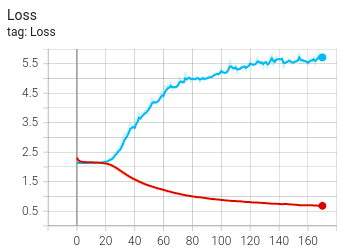# Is my multi-label classification model overfitting?

Hi, I am training a multi-label classifier. Looking at the loss and F1 curves, is my model overfitting?

I am using weighted BCE loss, along with dropout layers. What can I do to improve validation F1 and reduce validation loss, i.e., further prevent overfitting? Should I try `MultiLabelMarginLoss` for multi-label classification problem?

Appreciate any help on this.

(red is training, blue is validation)The model is definitely over-fitting, the validation curve should follow the trend of training curve, but the loss in this case increases, while the F1 Score is almost 0.
Can you please add the final layer of the model and the training script, along with the loss function being used to train the model. That might help in understanding how to reduce the validation loss and increase F1 score.

Thanks for your response. My multi-label classification model is as follows:

``````classifier = nn.Sequential(
nn.Conv1d(128, 128, 1),
nn.ReLU(),
nn.BatchNorm1d(128),
nn.Dropout(p=0.2),
nn.Conv1d(128, 128, 1),
nn.ReLU(),
nn.BatchNorm1d(128),
nn.Dropout(p=0.2),
nn.Conv1d(128, 1, 1)
)
``````

I’m making use of `BCEWithLogitsLoss` and I describe how I’m using it in this post: Loss for Multi-label Classifier

Please let me know how I can improve it.